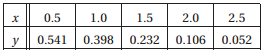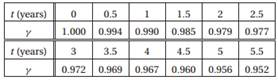Create an Account

Home / Questions / 1 Fit the function fx axebx to the data and compute the standard deviation 2 The inten...

1 Fit the function fx axebx to the data and compute the standard deviation 2 The intensity of radiation of a radioactive substance was measured at half year intervals The results were

1. Fit the function f(x) = axebx to the data and compute the standard deviation.2. The intensity of radiation of a radioactive substance was measured at half-year intervals. The results were:where γ is the relative intensity of radiation. Knowing that radioactivity decays exponentially with time: γ (t) = ae−bt, estimate the radioactive half-life of the substance.

Jul 27 2020 View more View LessSubscribe To Get Solution